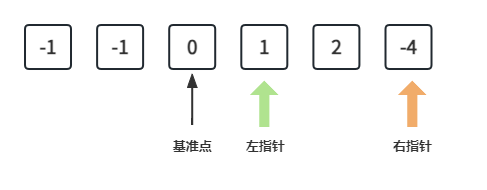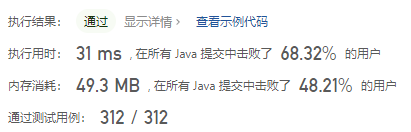#### 15. 三数之和

###### 来源: 每日一题 2023.07.09

``````输入：nums = [-1,0,1,2,-1,-4]

nums + nums + nums = (-1) + 0 + 1 = 0 。
nums + nums + nums = 0 + 1 + (-1) = 0 。
nums + nums + nums = (-1) + 2 + (-1) = 0 。

``````

``````输入：nums = [0,1,1]

``````

``````输入：nums = [0,0,0]

``````

``````3 <= nums.length <= 3000
-105 <= nums[i] <= 105
``````
``````class Solution {
public List<List<Integer>> threeSum(int[] nums) {

}
}
``````

#### 分析与题解

• 排序 + 双指针

这个题目我迷了.... 开始做的时候想往 `两数之和` 的Map缓存解法上靠拢,但是有一个问题就是 `两数之和` 实际上只有一个解法, 三数之和根据题意实际上会有多种解法,而且最重要的一点也是最不同的一点就是去重问题,以为当前题目会有重复元素,所以如何去重是必要的.

官方的解法是排序和双指针, 整体的解题思路是这样的.• 首先,我们需要做一些边界处理, 例如数组为空或者数组个数为0, 虽然题意已经帮我们明确这个不用处理....

``````if (length < 3 || nums == null) return result; // 边界
``````
• 然后使用Java的API `Arrays.sort` 对数组进行排序.

``````Arrays.sort(nums); // 排序
``````
• 排序完成之后,我们需要进行一次遍历,在遍历中寻找到第一个基准点,如上图所示.

• 寻找第一个基准点时,我们需要处理一下边界条件, 例如 `num[i] > 0``num[i] = num[i - 1]`

• 首先说一下为什么要处理 `num[i] > 0` . 其实如图所示, `num[i] > 0`时, 左右指针不管如何移动,三者之和必然大于0 (PS: 主要取决于左指针一开始的位置为 `num[i + 1]`), 所以这种情况下,数组后面的元素全部不用看了,肯定不满足题意了,直接就是 break.

• `num[i] = num[i - 1]` 这种情况主要是用来第一基准点的去重操作, num[i - 1] 有满足题意的组合,那么 num[i] 比如也会满足, 如果逻辑继续执行,必然会重复添加,所以这里需要进行去重操作

• 然后就是定义左右指针进行查看, 这里还是要注意这题有一个特殊点就是 左指针的起始位为 i + 1 , 如图所示.

• 在查看第二个基准点和第三个基准点时也需要去重操作,因为不去重的话,必然会造成重复的组合添加到最终结果中. 逻辑如下所示.

``````while(left < right && nums[left] == nums[left + 1]) left++;
while(left < right && nums[right] == nums[right - 1]) right--;
``````
• 其他情况这样按照双指针正常移动即可. 逻辑如下所示.

``````if (sum == 0) {
while(left < right && nums[left] == nums[left + 1]) left++;
while(left < right && nums[right] == nums[right - 1]) right--;
left++;
right--;
} else if (sum < 0) {
left++;
} else if (sum > 0) {
right--;
}
``````

最终,整个解题过程如下所示.

``````class Solution {
public List<List<Integer>> threeSum(int[] nums) {
List<List<Integer>> result = new ArrayList<List<Integer>>();
int length = nums.length;
if (length < 3 || nums == null) return result; // 边界
Arrays.sort(nums); // 排序

for (int i = 0; i < length; i++) {
// 边界: 当num[i] > 0 时, 就不用往下查看了,前面满足情况的就会带上nums[i]
// 只需要判断数组中找到的第一个数满足 nums[i] <= 0 情况即可.
// num[i] > 0 时, 左右指针不管如何移动,三者之和必然大于0
if (nums[i] > 0) break;
// 边界: 当 nums[i] = nums[i-1], 两者重复,查找情况就一致了,不需要重复查找
if (i > 0 && nums[i] == nums[i-1]) continue;
int left = i + 1;
int right = length - 1;
while(left < right) {
int sum = nums[i] + nums[left] + nums[right];
if (sum == 0) {
while(left < right && nums[left] == nums[left + 1]) left++;
while(left < right && nums[right] == nums[right - 1]) right--;
left++;
right--;
} else if (sum < 0) {
left++;
} else if (sum > 0) {
right--;
}
}
}
return result;
}
}
``````

复杂度分析:

• 时间复杂度: O(n²)
• 空间复杂度: O(㏒n)

结果如下所示.IT界无底坑洞栋主 欢迎加Q骚扰:676758285